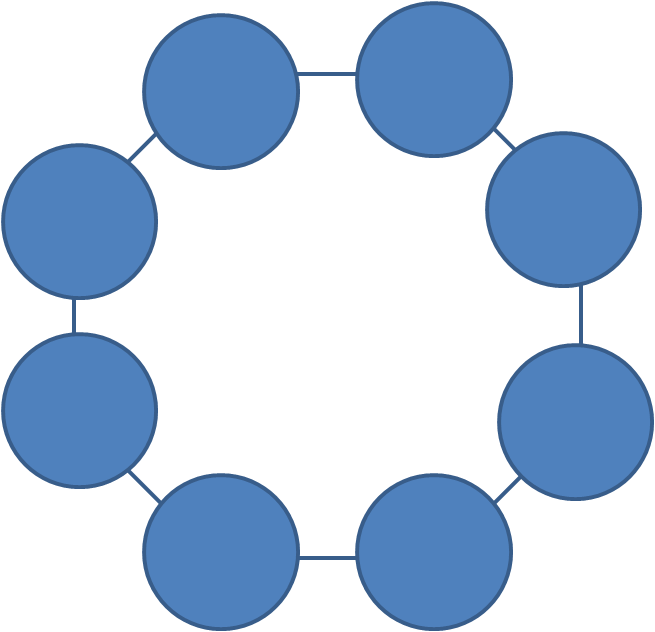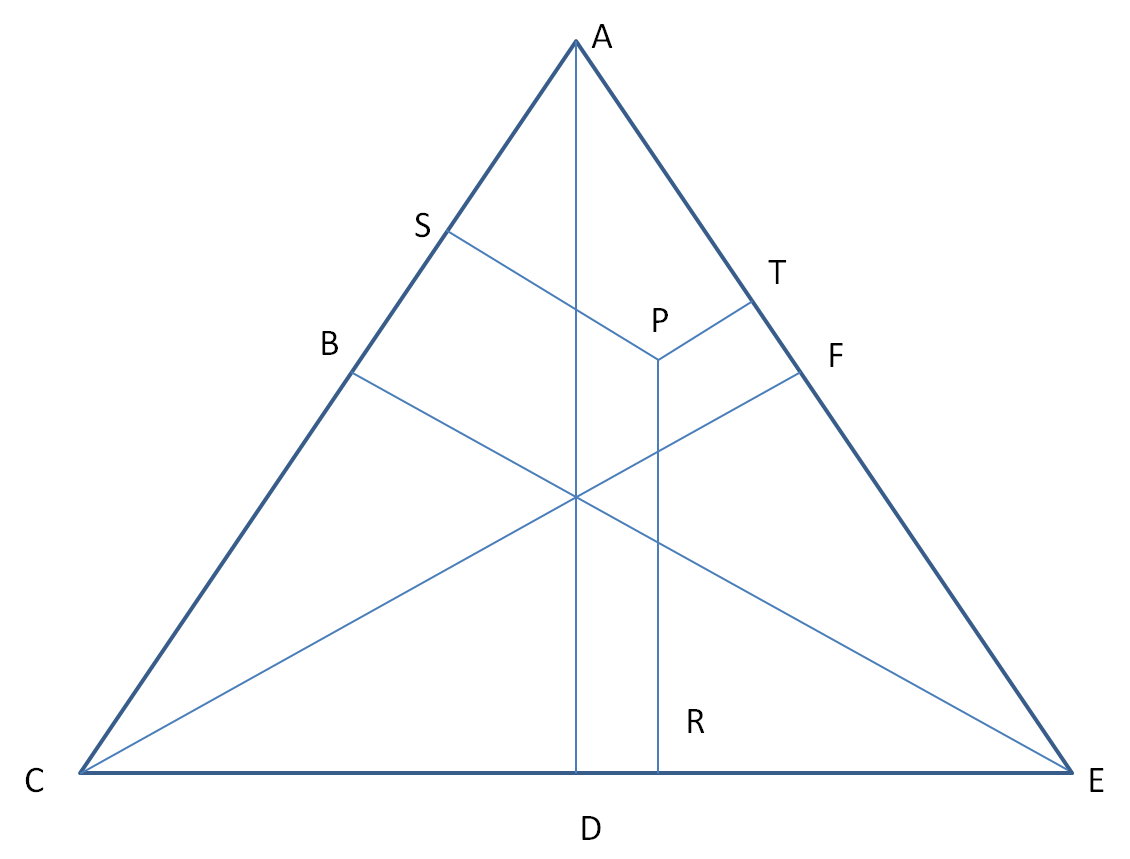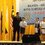# Tear the question paper, then throw it away

After the APMOPS, here are some questions that I translated:

1. A magician made a six-digit number $A$, and the digit sum of $A$ be $B$. The magician called out a spectactor to evaluate $A$-$B$. The spectactor said out 5 numbers, namely $0,2,4,6$ and $8$.The magician successfully revealed the last number. What number is it? Explain your reason.

*Bonus question: Find the minimum and maximum value for $A$.

1. The eight vertexes of an octagon are attached with circles, each required to fill up the numbers from 1 to 8. Can the sum of four consecutive attaching circles be:

a)larger than 16?

b) larger than 17?

If possible, find a way of doing so; if not, explain your reason.1. Wong cycled from station A to station B. Buses form station A and B each give out a bus at the same interval time (e.g. when station A gives out a bus every 30 minutes, station B does the same), but at different times. Every 6 minutes Wong meets up with a bus coming from the opposite, and every 9 minutes he is overtaken by a bus traveling at the same direction with him. It is known that all the buses from stations A & B take 50 minutes to travel to the other side ( it means that buses from station A travel 50 minutes to station B, and vice versa ). How long does it take for Wong to travel from station A to B?

4.In the figure below, 3 different heights of the triangle move from the bases to the vertexes of the triangle. P is a point in the triangle such that another 3 lines move from point P to the triangle, causing each of the lines to be parallel with AD, BE and CF respectively. If AD=2010 cm, BE= 2013 cm, CF = 2016 cm and PR = 1005 cm, PS= 671 cm, find the length of PT. (Oh, and I forgot, each line extending to the base is straight, or 90 degrees)Feel free to discuss! Enjoy!Note by Bryan Lee Shi Yang
6 years, 1 month ago

This discussion board is a place to discuss our Daily Challenges and the math and science related to those challenges. Explanations are more than just a solution — they should explain the steps and thinking strategies that you used to obtain the solution. Comments should further the discussion of math and science.

When posting on Brilliant:

• Use the emojis to react to an explanation, whether you're congratulating a job well done , or just really confused .
• Ask specific questions about the challenge or the steps in somebody's explanation. Well-posed questions can add a lot to the discussion, but posting "I don't understand!" doesn't help anyone.
• Try to contribute something new to the discussion, whether it is an extension, generalization or other idea related to the challenge.

MarkdownAppears as
*italics* or _italics_ italics
**bold** or __bold__ bold
- bulleted- list
• bulleted
• list
1. numbered2. list
1. numbered
2. list
Note: you must add a full line of space before and after lists for them to show up correctly
paragraph 1paragraph 2

paragraph 1

paragraph 2

[example link](https://brilliant.org)example link
> This is a quote
This is a quote
    # I indented these lines
# 4 spaces, and now they show
# up as a code block.

print "hello world"
# I indented these lines
# 4 spaces, and now they show
# up as a code block.

print "hello world"
MathAppears as
Remember to wrap math in $$ ... $$ or $ ... $ to ensure proper formatting.
2 \times 3 $2 \times 3$
2^{34} $2^{34}$
a_{i-1} $a_{i-1}$
\frac{2}{3} $\frac{2}{3}$
\sqrt{2} $\sqrt{2}$
\sum_{i=1}^3 $\sum_{i=1}^3$
\sin \theta $\sin \theta$
\boxed{123} $\boxed{123}$

Sort by:

Q1: The last number is $7$. $A$ can be expressed as $(10^5\times a+10^4\times b+10^3\times c+10^2\times d+10\times e+f)$ where $a,b,c,d,e$ and $f$ are the digits of $A$.

$B$ can be expressed as $(a+b+c+d+e+f)$.

Now, $A-B=99999a+9999b+999c+99d+9e$.

$A-B=9(11111a+1111b+111c+11d+e)$ implying that $A-B$ is a multiple of $9$.

Since, $A-B$ is a multiple of $9$, the digit sum of $A-B$ must also be divisible by $9$ (Divisibility Test of $9$).

Now, we are provided with $5$ digits of $A-B$ and we have to find the sixth one. The sum of these $5$ digits comes out to be $20$ and so for $A-B$ to be divisible by $9$, the sixth digit should be $7$ so that it sums out to be a multiple of $9$.

NOTE: There is a trick here. If the spectator would have called out $2,4,6,7$ and $8$ and then we were to find out the sixth digit, we could have $2$ different answers namely $0$ and $9$. Can you figure it out why?

- 6 years, 1 month ago

Because 2+4+6+7+8 IS a multiple of 9

- 6 years, 1 month ago

Yes, it has to be a multiple of $9$. This is true for all numbers and can be generalized easily for $n$-digit numbers.

- 6 years, 1 month ago

Q4 is 336.

- 2 years, 1 month ago

Q3 is 250 minutes.

- 2 years, 1 month ago